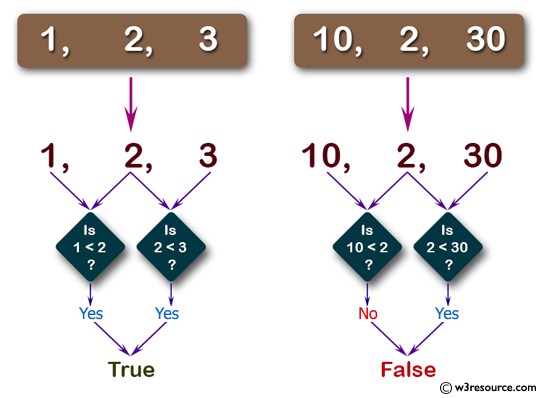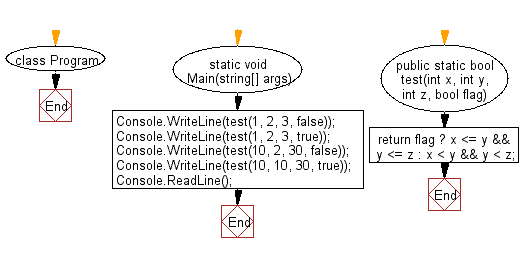﻿ C# - Check if three numbers are in strict increasing order# C# Sharp Basic Algorithm Exercises: Check whether three given numbers are in strict increasing order

## C# Sharp Basic Algorithm: Exercise-49 with Solution

Write a C# Sharp program to check whether three given numbers are in strict increasing order, such as 4 7 15, or 45, 56, 67, but not 4 ,8, 8 or 6, 6, 8.However,if a fourth parameter  is true, equality is allowed, such as 6, 6, 8 or 7, 7, 7.

Pictorial Presentation:Sample Solution:-

C# Sharp Code:

``````using System;
using System.Linq;
namespace exercises
{
class Program
{
static void Main(string[] args)
{
Console.WriteLine(test(1, 2, 3, false));
Console.WriteLine(test(1, 2, 3, true));
Console.WriteLine(test(10, 2, 30, false));
Console.WriteLine(test(10, 10, 30, true));
}
public static bool test(int x, int y, int z, bool flag)
{
return flag ? x <= y && y <= z : x < y && y < z;
}
}
}
```
```

Sample Output:

```True
True
False
True```

Flowchart:C# Sharp Code Editor:

Improve this sample solution and post your code through Disqus

What is the difficulty level of this exercise?

Test your Programming skills with w3resource's quiz.

﻿